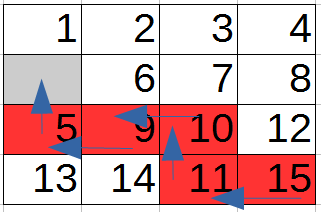2 views

I recently started an introductory course to Artificial Intelligence and I have been given the assignment to implement an admissible heuristic function in Python that solves the 15-Puzzle with A* search.

I implemented the Manhattan Distance along with some other heuristics. The Python code worked just fine and the algorithm solves the problem but I have some doubts as to whether the Manhattan distance heuristic is admissible for this particular problem.

According to theory, a heuristic is admissible if it never overestimates the cost to reach the goal. That means that the heuristic is optimistic and the cost it returns is never greater than the actual one.

When the initial state is the following (0 signifies the empty slot):

1  2 3  4

0  6 7  8

5  9 10 12

13 14 11 15

my program solves the problem with 5 moves, but the sum of the Manhattan Distances of every misplaced tile is equal to 10 which is double the value of the actual cost. So the real cost is much less than the estimated one. Does this mean that the heuristic is not admissible or is there something wrong with my logic?

I thought about counting just the empty block's Manhattan distance but that would lead to states with zero estimated costs when the empty block is in its correct place but other tiles are misplaced.

by (108k points)

The problem can be solved with five moves. Each time the empty tile is moved Up, Down, Right or Left. The five moves that resolve the problem are: Down, Right, Right, Down, Right. But if you employ the heuristic to the initial state it returns 10 which is double the actual cost. It should be less than the actual cost according to theory. You don't require the code to compute the Manhattan Distance.

In your example, the sum of the distance from the goal position of all tiles is 5 (tiles 5, 9, 10, 11, 15 need one move each).